You can Download Samacheer Kalvi 12th Maths Book Solutions Guide Pdf, Tamilnadu State Board help you to revise the complete Syllabus and score more marks in your examinations.

## Tamilnadu Samacheer Kalvi 12th Maths Solutions Chapter 9 Applications of Integration Ex 9.10

Choose the correct or the most suitable answer from the given four alternatives:

Question 1.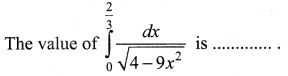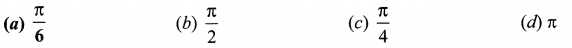Solution:
(a) $$\frac{\pi}{6}$$
Hint: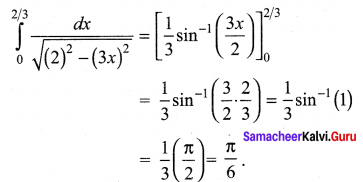Question 2.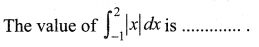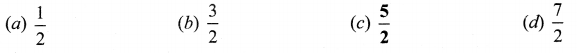Solution:
(c) $$\frac{5}{2}$$
Hint: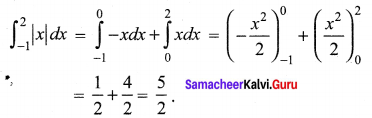Question 3.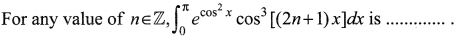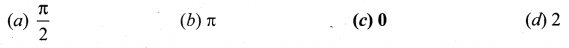Solution:
(c) 0
Hint: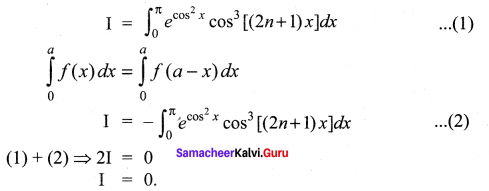Question 4.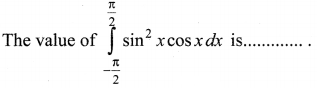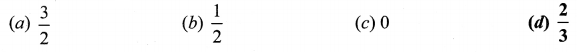Solution:
(d) $$\frac{2}{3}$$
Hint:
It is an even function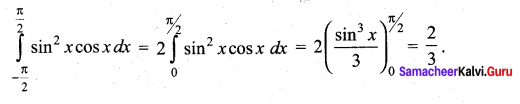Question 5.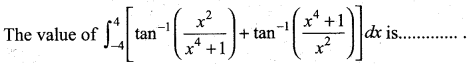(b) 2π
(c) 3π
(d) 4π
Solution:
(d) 4π
Hint: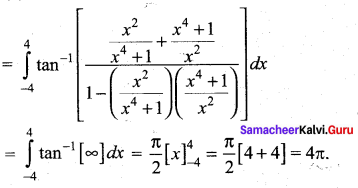Question 6.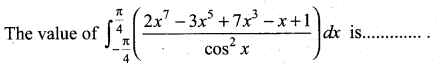(a) 4
(b) 3
(c) 2
(d) 0
Solution:
(c) 2
Hint: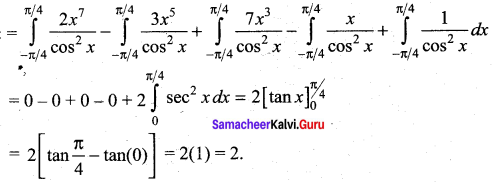Question 7.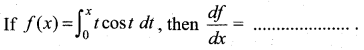(a) cos x – x sin x
(b) sin x + x cos x
(c) x cos x
(d) x sin x
Solution:
(c) x cos x
Hint: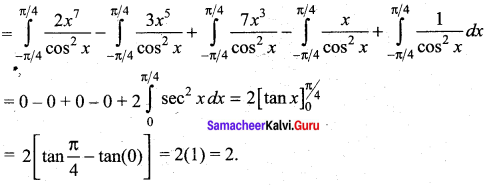Question 8.
The area between y2 = 4x and its latus rectum is ………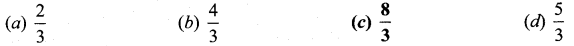Solution:
(c) $$\frac{8}{3}$$
Hint: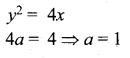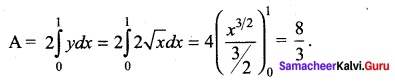Question 9.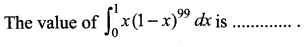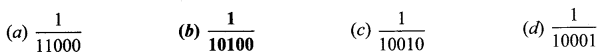Solution:
(b) $$\frac{1}{10100}$$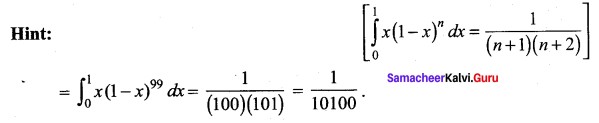Question 10.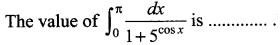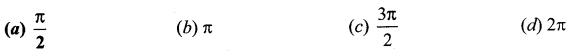Solution:
(a) $$\frac{\pi}{2}$$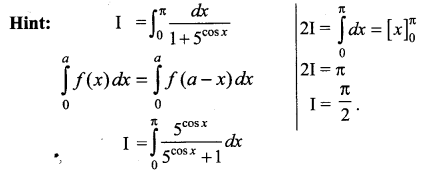Question 11.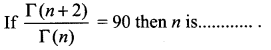(a) 10
(b) 5
(c) 8
(d) 9
Solution:
(d) 9
Hint: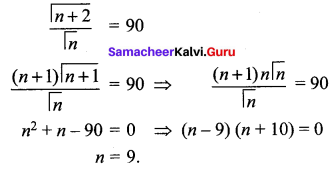Question 12.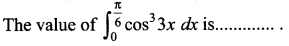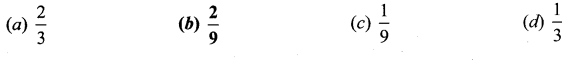Solution:
(b) $$\frac{2}{9}$$
Hint: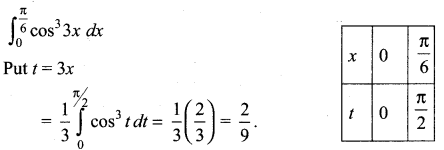Question 13.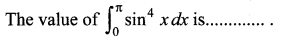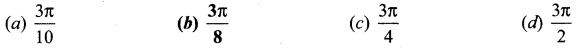Solution:
$$\frac{3 \pi}{8}$$
Hint: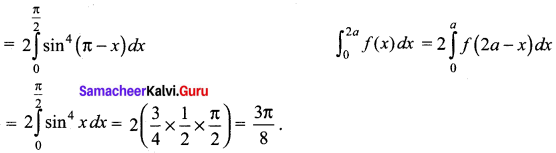Question 14.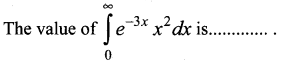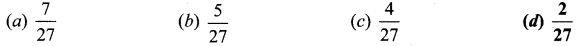Solution:
(d) $$\frac{2}{27}$$
Hint: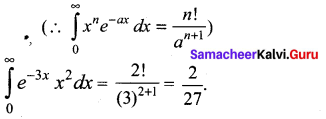Question 15.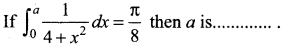(a) 4
(b) 1
(c) 3
(d) 2
Solution:
(d) 2
Hint: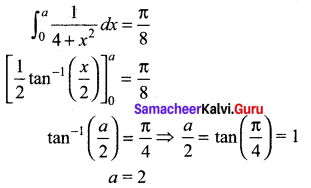Question 16.
The volume of solid of revolution of the region bounded by y2 = x(a – x) about x-axis is ……..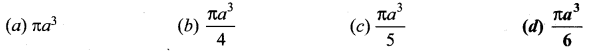Solution:
(d) $$\frac{\pi a^{3}}{6}$$
Hint: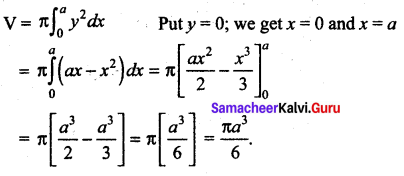Question 17.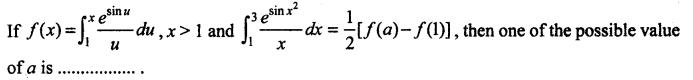(a) 3
(b) 6
(c) 9
(d) 5
Solution:
(c) 9
Hint: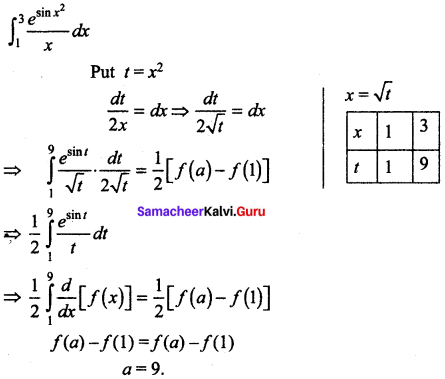Question 18.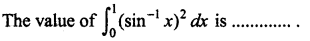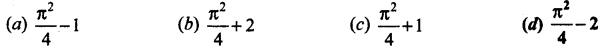Solution:
(d) $$\frac{\pi^{2}}{4}-2$$
Hint: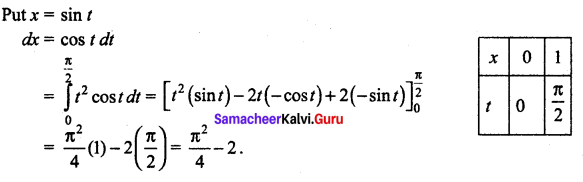Question 19.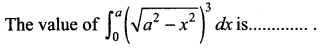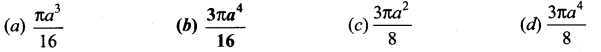Solution:
(b) $$\frac{3 \pi a^{4}}{16}$$
Hint: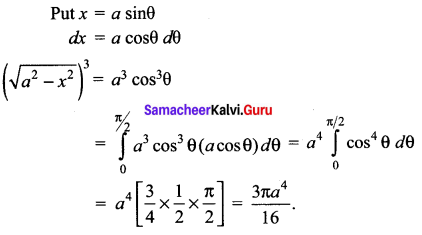Question 20.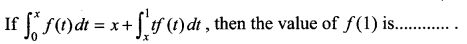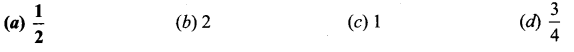Solution:
(a) $$\frac{1}{2}$$
Hint: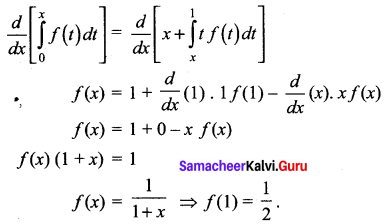### Samacheer Kalvi 12th Maths Solutions Chapter 9 Applications of Integration Ex 9.10 Additional Problems

Choose the correct or the most suitable answer from the given four alternatives:

Question 1.
The area bounded by the line y = x, the x – axis, the ordinates x = 1,x = 2 is …….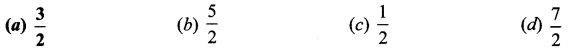Solution:
(a) $$\frac{3}{2}$$
Hint: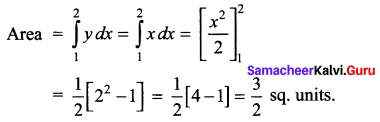Question 2.
The area of the region bounded by the graph of y = sin x and y = cos x between x = 0 and x = $$\frac{\pi}{4}$$ is ……..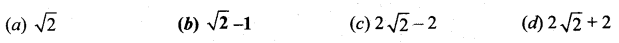Solution:
(b) $$\sqrt{2}-1$$
Hint: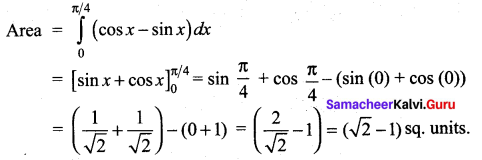Question 3.
The area between the ellipse $$\frac{x^{2}}{a^{2}}+\frac{y^{2}}{b^{2}}$$ = 1 and its auxiliary circle is …….
(a) πb(a – b)
(b) 2πa(a – b)
(c) πa(a – b)
(d) 2πb(a – b)
Solution:
(c) πa(a – b)
Hint: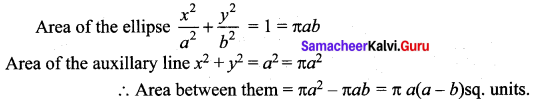Question 4.
The area bounded by the parabola y2 = x and its latus rectum is ……..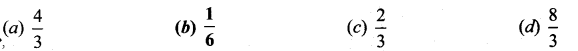Solution:
(b) $$\frac{1}{6}$$
Hint: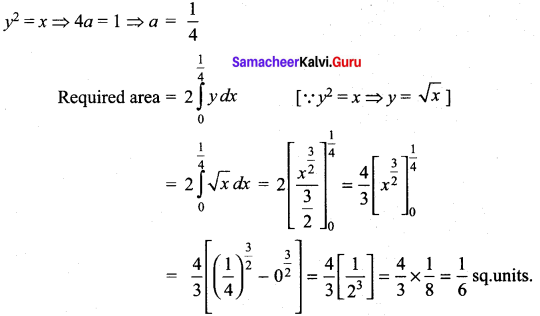Question 5.
The volume of the solid obtained by revolving $$\frac{x^{2}}{9}+\frac{y^{2}}{16}$$ = 1 about the minor axis is …….
(a) 48π
(b) 64π
(c) 32π
(d) 128π
Solution:
(b) 64π
Hint: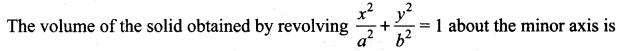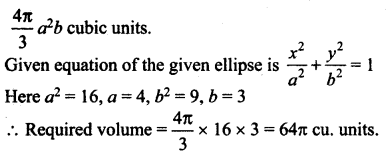Question 6.
The volume, when the curve y = $$\sqrt{3+x^{2}}$$ from x = 0 to x = 4 is rotated about x – axis is ……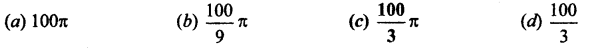Solution:
(c) $$\frac{100}{3} \pi$$
Hint: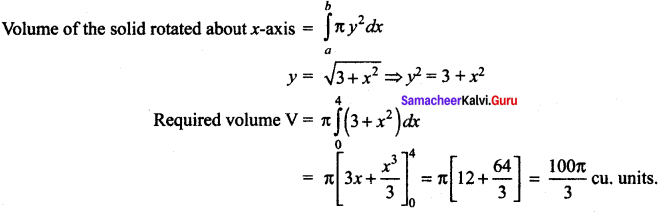Question 7.
The volume generated when the region bounded by y = x, y = 1, x = 0 is rotated about y – axis is ……….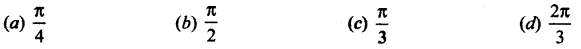Solution:
(c) $$\frac{\pi}{3}$$
Hint: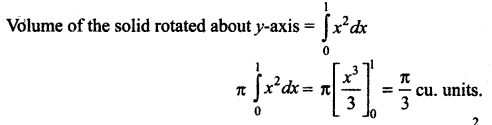Question 8.
Volume of solid obtained by revolving the area of the ellipse $$\frac{x^{2}}{a^{2}}+\frac{y^{2}}{b^{2}}$$ = 1 about major and minor axes are in the ratio …….
(a) b2 : a2
(b) a2 : b2
(c) a : b
(d) b : a
Solution:
(d) b : a
Hint: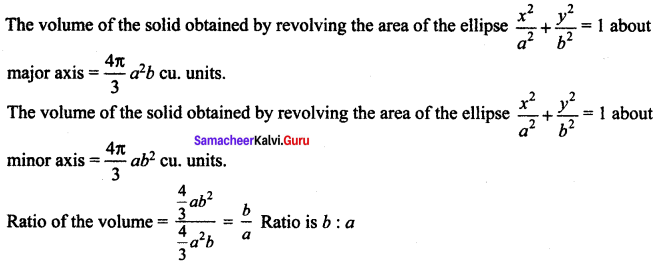Question 9.
The volume generated by rotating the triangle with vertices at (0, 0), (3, 0) and (3, 3) about x-axis is …….
(a) 18π
(b) 2π
(c) 36π
(d) 9π
Solution:
(d) 9π
Hint: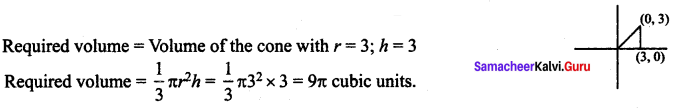Question 10.
The length of the arc of the curve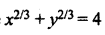is …….
(a) 48
(b) 24
(c) 12
(d) 96
Solution:
(a) 48
Hint:
Length of the arc of the curve = 6a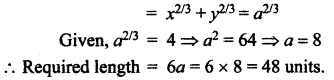∴ Required length = 6a = 6 × 8 = 48 units.Question 11.
The surface area of the solid of revolution of the region bounded by y = 2x, x = 0 and x = 2 about x-axis is ……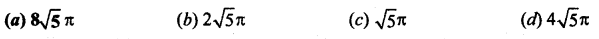Solution:
(a) $$8 \sqrt{5} \pi$$
Hint: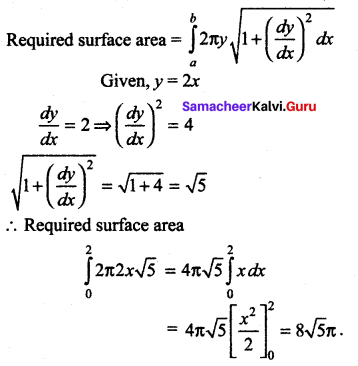Question 12.
The curved surface area of a sphere of radius 5, intercepted between two parallel planes of distance 2 and 4 from the centre is ……
(a) 20π
(b) 40π
(c) 10π
(d) 30π
Solution:
(a) 20π
Hint:
The curved surface area of a sphere of radius r intercepted between two parallel planes at a distance a and b from the centre of the sphere is 2πr (b – a)
Given radius, r = 5; a = 2; b = 4
Required surface area = 2πr (b – a)
= 2π × 5 × (4 – 2) = 20π sq. units